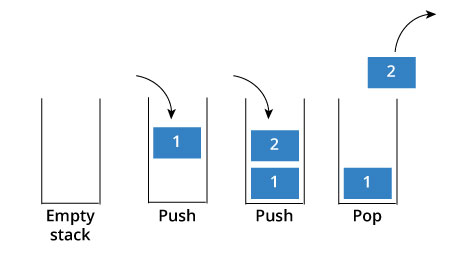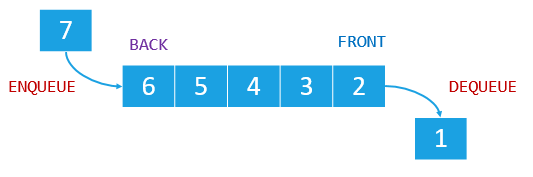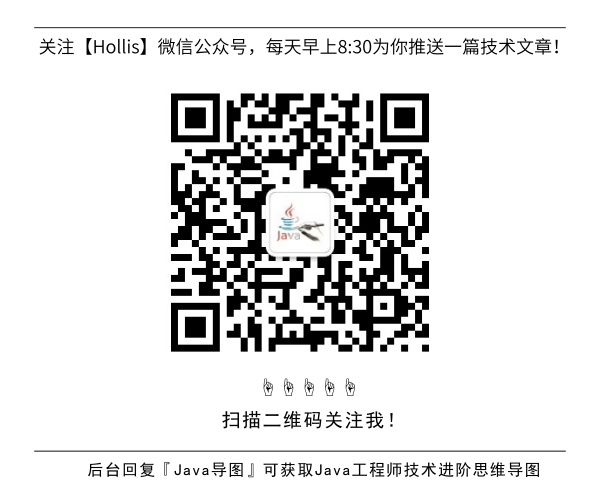# 我是一个栈

GitHub 24k Star 的Java工程师成神之路，不来了解一下吗！

### 数组### 链表### 我和我的胞弟### 爸爸妈妈是如何生出我和弟弟的

``````public class Stack<Item> implements Iterable<Item> {
private Item[] a;         // 数组表示栈，栈顶在最大的下标。
private int n;            // 栈内元素的个数

/**
* 初始化一个空栈
*/
public Stack() {
a = (Item[]) new Object;
n = 0;
}

/**
* 判断栈内是否有元素
*/
public boolean isEmpty() {
return n == 0;
}

/**
* 返回栈内元素个数
*/
public int size() {
return n;
}

// 改变栈的大小
private void resize(int capacity) {
assert capacity >= n;
// 注意不能直接创建泛型数组
Item[] temp = (Item[]) new Object[capacity];
for (int i = 0; i < n; i++) {
temp[i] = a[i];
}
a = temp;
// 也可以选择下面这种方式改变数组大小
// a = java.util.Arrays.copyOf(a, capacity);
}

/**
* 压入元素
*/
public void push(Item item) {
//先判断n的大小，如果栈满则改变栈的大小
if (n == a.length) resize(2*a.length);
a[n++] = item;
}

/**
* 弹出并返回元素
*/
public Item pop() {
if (isEmpty()) throw new NoSuchElementException("Stack underflow");
Item item = a[n-1];
a[n-1] = null;   //防止对象游离
n--;
// 如果有必要则调整栈的大小
if (n > 0 && n == a.length/4) resize(a.length/2);
return item;
}

/**
* 返回但不弹出栈顶元素
*/
public Item peek() {
if (isEmpty()) throw new NoSuchElementException("Stack underflow");
return a[n-1];
}

/**
* 返回一个可以进行先进后出迭代的迭代器
*/
public Iterator<Item> iterator() {
return new ReverseArrayIterator();
}

// 用内部类实现迭代器接口，实现从栈顶往栈底的先进后出迭代，没有实现remove()方法。
private class ReverseArrayIterator implements Iterator<Item> {
private int i;
public ReverseArrayIterator() {
i = n-1;
}
public boolean hasNext() {
return i >= 0;
}
public void remove() {
throw new UnsupportedOperationException();
}
public Item next() {
if (!hasNext()) throw new NoSuchElementException();
return a[i--];
}
}

/**
* 测试
*/
public static void main(String[] args) {
Stack<String> stack = new Stack<String>();
while (!StdIn.isEmpty()) {
if (!item.equals("-")) stack.push(item);
else if (!stack.isEmpty()) StdOut.print(stack.pop() + " ");
}
StdOut.println("(" + stack.size() + " left on stack)");
}
}
``````

``````public class Stack<Item> implements Iterable<Item> {
private Node<Item> first;     //栈顶节点
private int N;                // 栈内元素数量

// 辅助类Node，用于形成链表
private static class Node<Item> {
private Item item;
private Node<Item> next;
}

/**
* 初始化栈
*/
public Stack() {
first = null;
N = 0;
}

/**
* 判断栈是否为空
*/
public boolean isEmpty() {
return first == null;
//return N == 0;
}

/**
* 返回栈内元素数量
*/
public int size() {
return N;
}

/**
* 压入元素
*/
public void push(Item item) {
Node<Item> oldfirst = first;
first = new Node<Item>();
first.item = item;
first.next = oldfirst;
N++;
}

/**
* 弹出元素
*/
public Item pop() {
if (isEmpty()) throw new NoSuchElementException("Stack underflow");
Item item = first.item;        // 需弹出的元素
first = first.next;            // 删除头节点
N--;
return item;
}

/**
* 返回但不弹出元素
*/
public Item peek() {
if (isEmpty()) throw new NoSuchElementException("Stack underflow");
return first.item;
}

/**
* 从栈顶到栈底打印元素
*/
public String toString() {
StringBuilder s = new StringBuilder();
for (Item item : this)
s.append(item + " ");
return s.toString();
}

/**
* 实现Iterable接口
*/
public Iterator<Item> iterator() {
return new ListIterator<Item>(first);
}

// 实现Iterator接口用于迭代，没有实现remove方法
private class ListIterator<Item> implements Iterator<Item> {
private Node<Item> current;

//初始化时，current指向栈顶
public ListIterator(Node<Item> first) {
current = first;
}

public boolean hasNext() {
return current != null;
}

public void remove() {
throw new UnsupportedOperationException();
}

public Item next() {
if (!hasNext()) throw new NoSuchElementException();
Item item = current.item;
current = current.next;
return item;
}
}

/**
* 测试
*/
public static void main(String[] args) {
Stack<String> s = new Stack<String>();
while (!StdIn.isEmpty()) {
if (!item.equals("-")) s.push(item);
else if (!s.isEmpty()) StdOut.print(s.pop() + " ");
}
StdOut.println("(" + s.size() + " left on stack)");
}
}
``````

``````public class Queue<Item> implements Iterable<Item> {
private Item[] q;
private int N;          // 队列中元素的数量
private int first;      // 队头元素的下标
private int last;       // 队尾元素的后一个位置的下标，也就是元素入列时可以放置的位置

/**
* 初始化队列，此时头尾下标重合
*/
public Queue() {
q = (Item[]) new Object;
N = 0;
first = 0;
last = 0;
}

/**
* 依旧用N判断队列是否为空
*/
public boolean isEmpty() {
return N == 0;
}

/**
* 队列中元素数量
*/
public int size() {
return N;
}

// 调整数组大小
private void resize(int max) {
assert max >= N;
Item[] temp = (Item[]) new Object[max];
//注意这里：把N个元素放入总大小为max的队列（max>=N）
//因为循环使用数组，从first开始的第i个元素可能保存在了first
//前面（即last在first前面）。
for (int i = 0; i < N; i++) {
temp[i] = q[(first + i) % q.length];
}
q = temp;
//把小队列按顺序复制到大队列后重置队头和队尾
first = 0;
last  = N;
}

/**
* 元素入列
*/
public void enqueue(Item item) {
if (N == q.length) resize(2*q.length);
q[last++] = item;   // 元素入列
if (last == q.length) last = 0;  // 如果last超出数组下标，把last置零，循环利用数组
N++;
}

/**
* 元素出列
*/
public Item dequeue() {
if (isEmpty()) throw new NoSuchElementException("Queue underflow");
Item item = q[first];
q[first] = null;       // 防止对象游离
N--;
first++;
if (first == q.length) first = 0; // 循环利用数组，下一个队头在下标为0的地方
if (N > 0 && N == q.length/4) resize(q.length/2);
return item;
}

/**
* 返回队头元素但不出列
*/
public Item peek() {
if (isEmpty()) throw new NoSuchElementException("Queue underflow");
return q[first];
}
/**
* 实现Iterable接口
*/
public Iterator<Item> iterator() {
return new ArrayIterator();
}

//实现迭代器
private class ArrayIterator implements Iterator<Item> {
//维护一个i用于迭代
private int i = 0;
public boolean hasNext()  { return i < N; }
public void remove()      { throw new UnsupportedOperationException();  }

//直接利用first进行遍历，注意可能存在数组的循环利用
public Item next() {
if (!hasNext()) throw new NoSuchElementException();
Item item = q[(i + first) % q.length];
i++;
return item;
}
}

/**
* 测试
*/
public static void main(String[] args) {
Queue <String> q = new Queue <String>();
while (!StdIn.isEmpty()) {
if (!item.equals("-")) q.enqueue(item);
else if (!q.isEmpty()) StdOut.print(q.dequeue() + " ");
}
StdOut.println("(" + q.size() + " left on queue)");
}

}
``````

``````public class Queue<Item> implements Iterable<Item> {
private Node<Item> first;    // 队头节点
private Node<Item> last;     // 队尾节点（注意和上面的last区分，last并不是队尾元素的下标）
private int N;               // 队列元素的数量

// 辅助类Node
private static class Node<Item> {
private Item item;
private Node<Item> next;
}

/**
* 初始化队列
*/
public Queue() {
first = null;
last  = null;
N = 0;
}

public boolean isEmpty() {
return first == null;
}

public int size() {
return N;
}

/**
* 返回但不删除头元素
*/
public Item peek() {
if (isEmpty()) throw new NoSuchElementException("Queue underflow");
return first.item;
}

/**
* 元素入列
*/
public void enqueue(Item item) {
//记录尾节点
Node<Item> oldlast = last;
//创建新的尾节点
last = new Node<Item>();
last.item = item;
last.next = null;
//如果队列是空的，将first置为last，因为这时候队列中只有一个元素
if (isEmpty()) first = last;
//否则执行正常的在尾节点插入新节点的操作
else           oldlast.next = last;
N++;
}

/**
*元素出列
*/
public Item dequeue() {
if (isEmpty()) throw new NoSuchElementException("Queue underflow");
//队头元素出列
Item item = first.item;
first = first.next;
N--;
//如果这时候队列为空，表示原来只有一个元素，这时候也将last置为null
if (isEmpty()) last = null;
return item;
}

public String toString() {
StringBuilder s = new StringBuilder();
for (Item item : this)
s.append(item + " ");
return s.toString();
}
public Iterator<Item> iterator()  {
return new ListIterator<Item>(first);
}

// 实现迭代
private class ListIterator<Item> implements Iterator<Item> {

private Node<Item> current;
//要实现迭代，我们只需要维护一个节点，并在开始的时候将它置为first
public ListIterator(Node<Item> first) {
current = first;
}

public boolean hasNext()  { return current != null;}
public void remove()      { throw new UnsupportedOperationException();  }

public Item next() {
if (!hasNext()) throw new NoSuchElementException();
Item item = current.item;
current = current.next;
return item;
}
}

/**
* 测试
*/
public static void main(String[] args) {
Queue<String> q = new Queue<String>();
while (!StdIn.isEmpty()) {
if (!item.equals("-")) q.enqueue(item);
else if (!q.isEmpty()) StdOut.print(q.dequeue() + " ");
}
StdOut.println("(" + q.size() + " left on queue)");
}
}
``````

### 参考资料

“数组、堆栈”与“链表、队列”的区别

(全文完)## HollisChuang's Blog

• 微信咨询
• 去评论
• 回顶
###### 回顶部